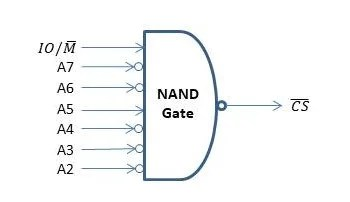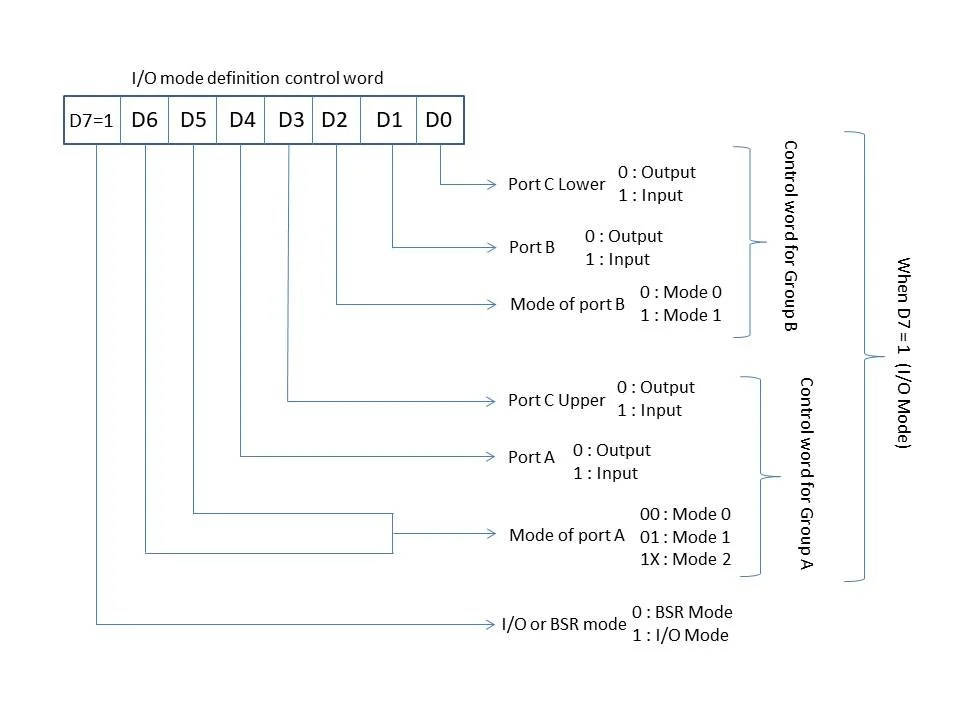View Course Path

# Interfacing of 8085 with 8255 Programmable Peripheral Interface

The 8255 is a programmable peripheral interface. It is an IC used to simplify the interfacing of microprocessors and microcontrollers with I/O devices and increase the number of I/O devices that can be interfaced. The best part is that it is programmable, which means that we don’t have to change circuit connections and wiring in order to switch between different ports and modes of operation.

Make sure you understand the working of the 8255 before you begin this tutorial. You can have a look at our in-depth guide on the working of the 8255 to learn more about how it works and the different operating modes. In this post, we will be covering the interfacing of 8255 with the 8085 microprocessor, and we will take up a simple example of taking two numbers as inputs, adding them, and then sending the result to the output port.

Contents

## Circuit diagram to connect the 8085 with 8255You can understand the connections in the following steps.

• First of all, we need supply power to both 8085 and 8255 by connecting VCC and GND pins to the appropriate sources.
• RESET OUT pin of 8085 is connected to the RESET pin of 8255. When the microprocessor is reset, it also resets the 8255 via this connection.
• RD* and WD* pins of 8085 are connected to the RD* and WD* pins of 8255, respectively. 8085 conveys the type of operation (read or write) to 8255 via these connections.
• As we have come to know in this 8085 course, pins AD0-AD7 have dual functionality. They act as both; address bus and data bus. This is achieved by multiplexing. To extract address from it, we need to demultiplex it, which is achieved using the IC 74LS373. To learn in detail about the demultiplexing process, you can check out this section on demultiplexing AD0-AD7.
• After demultiplexing, pins A0 and A1 (from the output of IC74LS373) are connected to the pins A0 and A1 of 8255, respectively. These two pins tell 8255 about which port the microprocessor is talking about.
• The last part of making the connections is the chip select logic.

### Chip select logic

For the microprocessor to be able to communicate with 8255, the CS* (active low chip select signal) should be low. So, we design a logic circuit that takes address lines AD2-AD7 as inputs and CS* signal as output. The design should be such that if the address allotted to any of the ports A, B, or C appears on AD0-AD1, output CS* should go low.

Let us allot the address to the ports A, B, and C of 8255.

• Port A: 0010 0000 (20H)
• Port B: 0010 0001 (21H)
• Port C: 0010 0010 (22H)
• Control port: 00100011 (23H)

The last two bits are A0 and A1. The rest of the 6 bits are used to generate chip select signals.

Note: We are taking only the lower eight bits of the address (AD0-AD7) into consideration because here, we define the ports A, B, and C as I/O mapped I/O and not memory-mapped I/O.

• I/O mapped I/O has an 8-bit address.
• Memory-mapped I/O has a 16-bit address.

Brush up your concepts on the difference between I/O mapped I/O and memory-mapped I/O here.

From the above address allocations, we can see that CS* should be low only when A7 = A6 = A4 = A3 = A2 = 0 and A5 = 1.

Since we are using the NAND gate to implement chip select logic, we should represent the boolean expression in the sum of product form.

CS = Complement of ( A7 + A6 + A5 + A4 + A3 +  A2 )

So, the final circuit of the chip select logic will be## Algorithm

Consider this example where we are required to take input data from port A and port B and output it at port C. Let’s solve this problem step by step.

Step 1

Specify the control word and send it to the control port. The control word of 8255 in I/O mode is shown in the illustration below.• Ports will be working in I/O mode and not in BSR mode. So, D7=1.
• This is a simple I/O operation. So, we need all three ports A, B, and C to work in mode 0. So, D6 = D5 = D2 = 0.
• Port A and B are in input mode and port C is in output mode. Therefore, D4 = D1 = 1 and D3 = D0 = 0.

The control word would be 1001 0010 in binary and 92H in hexadecimal. Now, we write this data to the control port to set the port into desired modes. This completes step 1.

Step 2

Read input from port A. Input will be stored in the accumulator. But we need to read another input from port B, and hence, we move this data to register C.

Step 3

Step 4

Now, we have the two numbers to be added in Accumulator and register C. We add both of them and output the value to port C.

Let’s translate the above steps into assembly language code.

## Assembly language program to interface 8255 with 8085

```MVI A, 92H    ;Load the control word into the accumulator
OUT 23H       ;Write the control word the the control port of 8255
MOV C, A      ;Move the input from port A to register C to make room
;for the input data from port B
OUT 22H       ;Output the result of addition to port C with address 22H
```

If you have any questions or want to discuss further regarding this topic, leave a comment below and we will get back to you.

## 5 thoughts on “Interfacing of 8085 with 8255 Programmable Peripheral Interface”

1.ADS says:

This course is really helpful.I want to know the program for generating a square wave.Thanks in advance.

2.hepl says:

Assume that port B 8255A PPI is the source for 256 bytes of data to 8085. Write assembly code to receive data from port B of 8255A PPI with a delay of 500 ms. After that, save the received data at memory locations starting at 2000H.Can any help me

3.Help says:

Assume that port B 8255A PPI is the source for 256 bytes of data to 8085. Write assembly code to receive data from port B of 8255A PPI with a delay of 500 ms. After that, save the received data at memory locations starting at 2000H.
I get this question, can u help me how to write for delay.

4.Helpingly says:

Why ALU operation is not possible with i/o data in peripheral(i/o) mode in 8085,wherease incase of memory mapped mode ALU operation are possible on i/o data ?
doubt came after reading Ramesh gaonkar book of 8085(difference between memory mapped i/o and peripheral i/o)
thank u

1.Umair Hussaini says:

That’s a good question actually. The answer is really simple but it threw me off for a bit haha! So the data in I/O mode moves between the peripheral device (the port address) and Accumulator. Check the arithmetic instructions for 8085. Also, check the logical instructions for 8085. You will notice that there are no direct instructions to perform arithmetic or logical operations with data stored at a port address. Arithmetic and logical instructions are available only between the accumulator and registers/register pairs/direct data. And in I/O mode we only have IN and OUT instructions. Hence, for any arithmetic or logical operation to be performed on data from peripherals, we first have to use the IN command, get the data into the ALU, then move it to a register and then perform the operation. So to answer your question in short. ALU operations are not possible with I/O data in peripheral mode simply because the system is not designed for direct manipulation of the I/O data. You have to jump through a few hoops to do it. I hope that answers your question. Good question! I will add this to our post on the difference between I/O mapped I/O and memory-mapped I/O.

This site uses Akismet to reduce spam. Learn how your comment data is processed.